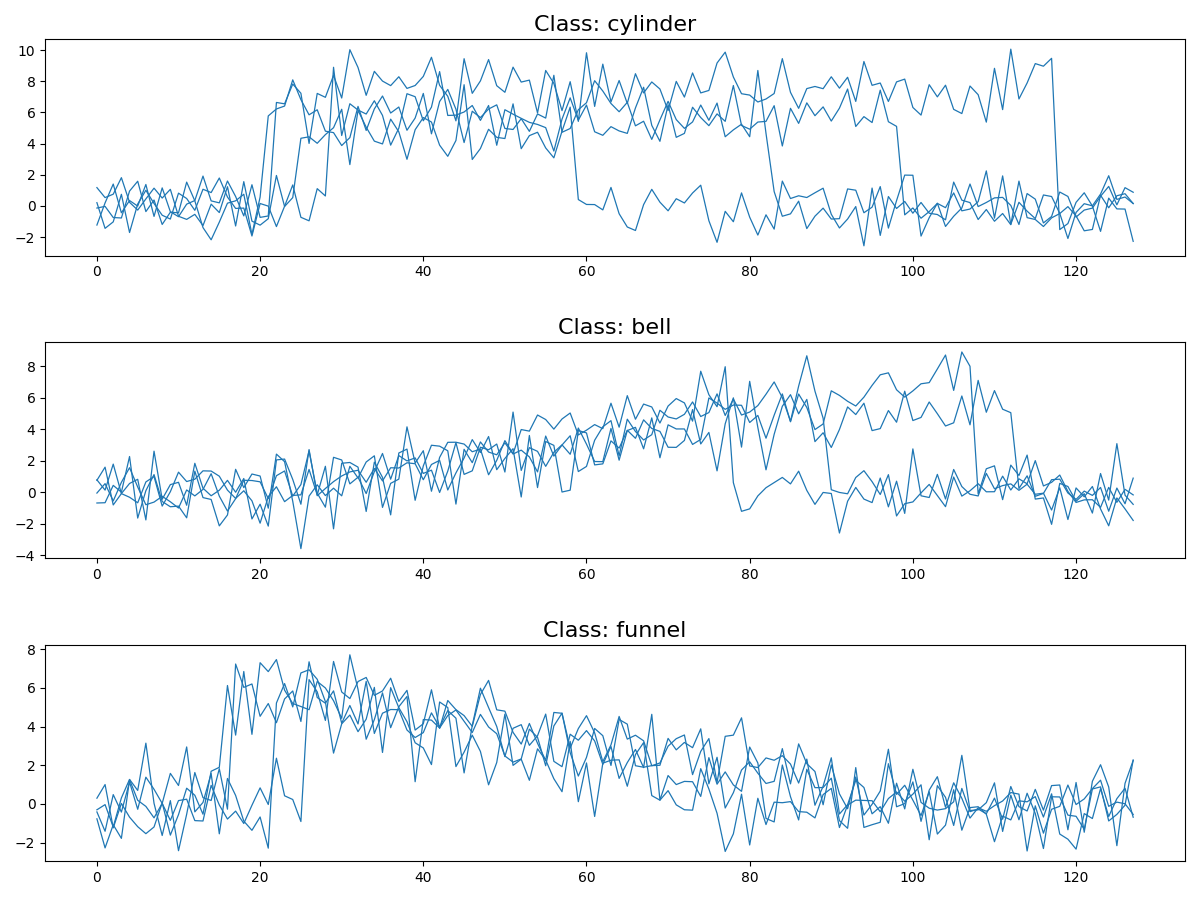# Making a Cylinder-Bell-Funnel dataset¶

This example shows how to generate a Cylinder-Bell-Funnel dataset. This simulated dataset was introduced by N. Saito in his Ph.D. thesis entitled “Local feature extraction and its application”. It is one of the most well-known datasets in time series classification. It is implemented as `pyts.datasets.make_cylinder_bell_funnel()`.```# Author: Johann Faouzi <johann.faouzi@gmail.com>

import matplotlib.pyplot as plt
from pyts.datasets import make_cylinder_bell_funnel

X, y = make_cylinder_bell_funnel(n_samples=12, random_state=42)

plt.figure(figsize=(12, 9))
for i, classe in enumerate(['cylinder', 'bell', 'funnel']):
plt.subplot(3, 1, i + 1)
for x in X[y == i]:
plt.plot(x, color='C0', linewidth=0.9)
plt.title('Class: {}'.format(classe), fontsize=16)

plt.tight_layout()